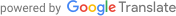### Blog

### A patenting strategy derived from a simulated experience of artificial intelligence development 2.

Blog > テクノロジー > A patenting strategy derived from a simulated experience of artificial intelligence development 2.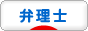The first entry is here.

In the first entry, we showed that we could estimate the specification authors with high precision in a simulated developed neural network. In the development process, the neural network learned based on the following three patterns of input data.
1. Habit of document expression.
2. Habit of flow of logic.
3. Both habit of document expression and Habit of flow of logic.
Below, I will concretely describe each pattern.

1. Habit of document expression.
This is the plan mentioned in the 1st entry. In this plan, the following 11 items were digitized for each specification before learning. Number of lines of main claim, number of characters of main claim, average number of lines of subclaims, average number of characters of subclaims, number of commas per line of claims, number of characters per line of claims, average number of commas per paragraph of the specification, average number of characters per paragraph of the specification, number of commas per specification, number of characters per specification, the number of characters of the specification.
Details of learning are as follows.
· Training data: 300 data correlating the numerical values of 11 items with the author.
· Test data: 90 data correlating the numerical values of 11 items and the author.
· Hidden layer: One layer with 7 nodes.
· Mini batch: about 10 to 50.
· Optimization method: stochastic gradient descent.
· Iteration: 50000 times. By doing the above learning with training data and estimating 90 authors of test data in the neural network after learning, the correct answer rate was 88% (Fig. 1).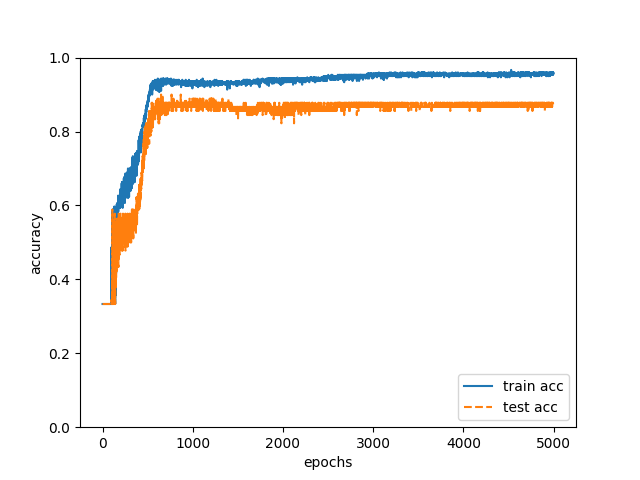Fig. 1

2. Habit of flow of logic.
In this plan, we measured the number of seven words below for each specification before learning.
“However”, “still”, “namely”, “also”, “further”, “could be “, “and”.
I often write sentences in the description in the order of “assertions, commentaries, arguments, and exemplification” in order to explain logically. When writing sentences in this order, “namely” appears before “commentary”. Since the habit of logical explanation differs for each author, I thought that the authors can be identified by statistically processing the words in the first part and the last part of the paragraph. The details of learning are the same as “1. Habit of document expression” (however, the number of nodes in the hidden layer is five). By doing the above learning with training data and estimating 90 authors of test data, the correct answer rate was 79% (Fig. 2).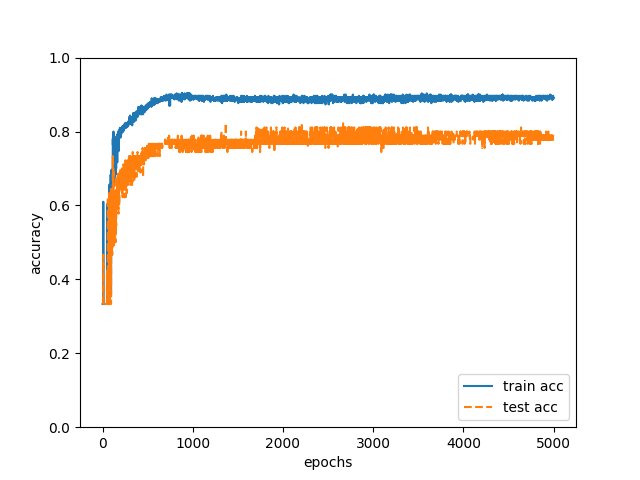Fig. 2

As described above, the correct answer rate of 79% was obtained when “1. Habit of document expression ” was used as input data, and the correct answer rate of 88% was obtained when “2. Habit of flow of logic” was used as input data. This result was different from my intuition, so I was a little puzzled. If I estimate authors by myself, I pay attention to logic flow more than the number of punctuation marks.
Although “2. Habit of flow of logic” may have disadvantage because the number of items is small, I can say that “1. Habit of document expression ” is effective to estimate the authors. Based on this result it is impossible to exclude the idea that “1. Habit of document expression” can be used as input data if seriously applying for a patent.
Furthermore, although the percentage of correct answers is somewhat reduced, the correct answer rate of 79% can be obtained even in “2. Habit on flow of logic “, so if you apply for a patent, applicant should not omit “2. Habit of flow of logic ” from claims.
A significant correct answer rate was obtained when one of “1. Habit of document expression” and “2. Habit of flow of logic” was used as input data, so it will be possible to estimate more accurately if both are set as input data.

Therefore, I let the neural network learn based on “3. Both habit of document expression and habit of flow of logic”. We created training data and test data by combining the input data used in “1. Habit of document expression” and “2. Habit of flow of logic”. The detail of learning is the same as “1. Habit of document expression” (nodes of the hidden layer are 11). We conducted the above training with training data and estimated the authors of 90 test data in the neural network after learning, the correct answer rate was 93% (Fig. 3). 84 of the 90 test data have been able to accurately estimate the author.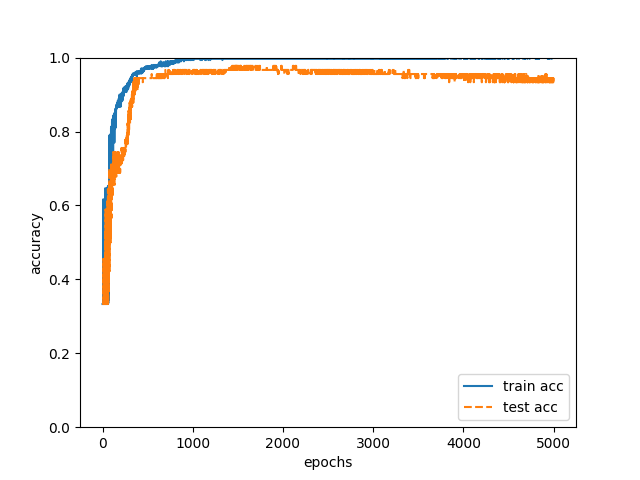Fig. 3

It was proved to be effective that “1. Habit of document expression” “2. Habit of flow of logic” “3. Both habit of document expression and habit of flow of logic ” are very useful as input data for estimating the author of the specification. On the other hand, in developing neural networks, it is necessary to optimize the structure of the network. Next time we will report the result of changing the structure of the network.

The third entry is here.
The fourth entfy is here.